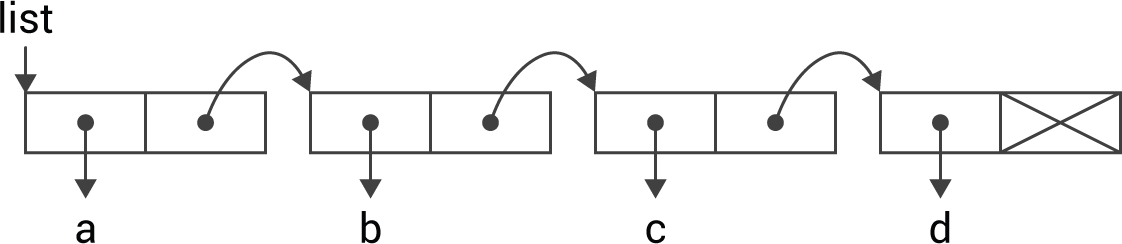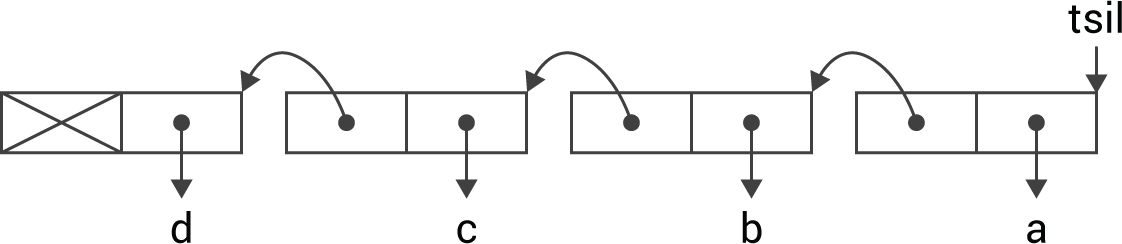## Lisp through the looking-glass

26th November 2017

The most fundamental construct in Lisp is the list, implemented as a linked list of cons cells, represented in every Lisp textbook with a diagram like this:which, of course, is the internal representation for the list:

`(a b c d)`

### Looking-Glass Lisp

However, Lisp could equally well have been implemented with the lists going right to left, rather than left to right. So in Looking-Glass Land the internal representation for this list would be:In normal Lisp this has the representation:

`((((nil . d) . c) . b) . a)`

Exploring this a bit further, let's write the Looking-Glass equivalents of first, rest, and cons. Initially I decided to call these tsrif, tser, and snoc, but that soon became a bit annoying, so I settled on ~first, ~rest, and ~cons.

Here are the definitions:

```(defun ~first (list) (cdr list))
(defun ~rest (list) (car list))
(defun ~cons (a d) (cons d a))```

Next, here's a function ~ to convert a list in normal Lisp representation to Looking-Glass representation:

```(defun ~ (list)
(cond
((null list) nil)
(t (cons (~ (cdr list)) (car list)))))```

For example:

```CL-USER > (~ '(a b c d))
((((nil . d) . c) . b) . a)```

For convenience, we can define { and } as reader macros to represent Looking-Glass lists:

```(set-macro-character #\{ '~list-reader)
(set-macro-character #\} (get-macro-character #\) nil))

(declare (ignore char))

This defines { as a macro character that calls ~list-reader, and } as a second macro character that behaves like ). So now we can write:

```CL-USER > '{a b c d}
((((nil . d) . c) . b) . a)```

### Longest Common Subsequence

Finally, to prove that this alternative Looking-Glass representation works just as well as the standard representation, here's a Looking-Glass version of the recursive Longest Common Subsequence routine I presented in the earlier article Longest Common Subsequence. It finds the longest sequence of elements that occur in the same order in both sequences, and is called ~lcs.

First we need to define ~length:

```(defun ~length (list)
(cond
((null list) 0)
(t (1+ (~length (~rest list))))))```

Then here's the definition of ~lcs:

```(defun ~longest (a b)
(if (> (~length a) (~length b)) a b))

(defun ~lcs (a b)
(cond
((or (null a) (null b)) nil)
((eql (~first a) (~first b))
(~cons (~first a) (~lcs (~rest a) (~rest b))))
(t (~longest (~lcs a (~rest b)) (~lcs (~rest a) b))))) ```

Trying it out:

```CL-USER > (~lcs '{1 2 3 5 6 8 9} '{2 3 1 4 6 7 8})
((((nil . 8) . 6) . 3) . 2)```

In other words,

`{2 3 6 8}`